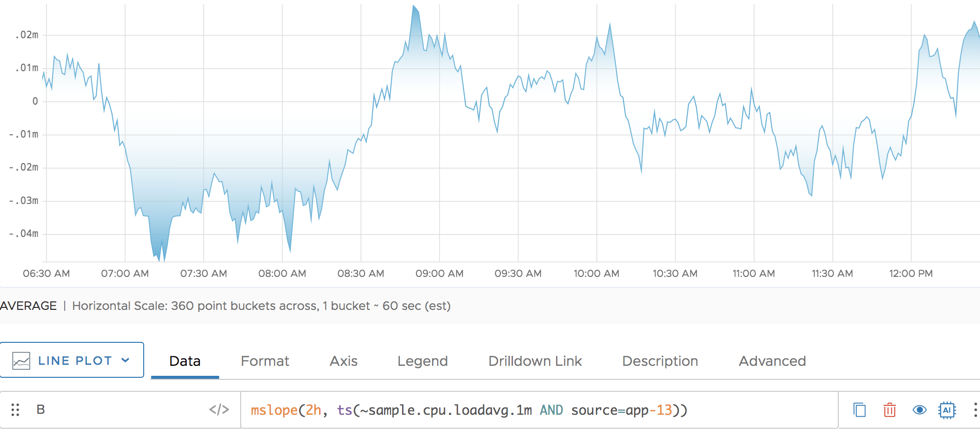Reference to the mslope() function

## Summary

``````mslope(<timeWindow>, <tsExpression>)
``````

Per-second derivative of the linear regression of the time series over the specified time window.

ParameterDescription
timeWindow Amount of time in the moving time window. You can specify a time measurement based on the clock or calendar (1s, 1m, 1h, 1d, 1w), the window length (1vw) of the chart, or the bucket size (1bw) of the chart. Default is minutes if the unit is not specified.
tsExpression Optional expression to which you want to apply this function.

## Description

This moving time window function is a measure of how quickly a metric is changing. The function uses simple linear regression over a time window to calculate the per-second derivative.

## Example

The following screenshot shows the result of `mslope()` for a simple metric.